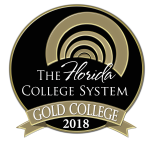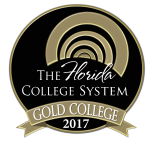MAC2312

## Calculus and Analytic Geometry II

Course Code:
MAC2312

Credit Hours:
4

Effective beginning:
2021-22

Course Description:
This is a course which includes techniques of integration; applications of the integral; polar coordinates; sequences and series; Taylor series; conic sections; and vectors. A “C” grade or higher must be earned to advance to a higher level mathematics course or to satisfy part of the general education requirement in mathematics.

### Course Details

Prerequisites:

A “C” grade or higher in MAC 1311

##### Instructors:

Stan Young
youngs1@chipola.edu

##### Required textbooks/ course materials:

Calculus, 11th Edition, by Larson & Edwards, Brooks/Cole, Cengage Learning

Enhanced WebAssign Printed Access Card for Calculus, Multi Term Courses ISBN: 9781337652650 OR
Text + Enhanced WebAssign Homework and eBook LOE Printed Access Card for Multi Term Math and Science ISBN: 9781337604741

A TI-84 graphing calculator is required for this course.

##### Assignment/course outline:

See your Instructor First Day Handout for individual instructor assignment schedule.

##### Discipline-level learning outcomes:

Demonstrate Basic Mathematical Skills and Knowledge

M-1  Apply arithmetic, algebraic, or geometric skills to solve mathematical problems.

M-2  Represent basic mathematical information verbally, numerically,  graphically, or symbolically.

M-3  Solve mathematical problems using appropriate technology.

M-4  Interpret mathematical models  such as formulas, graphs, tables and schematics.

M-5  Use mathematical processes to solve contextual problems.

##### Course-level learning outcomes:

Course-level student learning outcomesDiscipline-level learning outcomesAssessment methods

Evaluate definite, indefinite, and improper integrals by using basic integration rules, integration by parts, trigonometric integrals, trigonometric substitution, partial fractions, and integration tables.

Construct Maclaurin and Taylor polynomials and bound the error in using such a polynomial to approximate a function.

Test for convergence of an infinite series using the comparison, limit comparison, ratio, root, divergence, alternating series, and integral tests, and use the sequence of partial sums in determining convergence.

Construct a power series representation for a function, and evaluate where it converges.

Identify conics by their equations and/or properties, use polar coordinates and polar graphs, and write parametric equations for plane curves.

Identify points in space, calculate the distance between points, find the equation, center, and radius of a sphere, and perform basic operations of vectors in the plane and in space.

M1, M2, M3, M4

M1, M2, M3, M4

M1,M2,M3, M4

M1,M2,M3, M4

M2, M3, M4

M1, M2, M3, M4, M5

Unit Tests, Cumulative Final, Homework, Problem Solving

##### Means of accomplishing learning outcomes:

Teacher Facilitated: The teacher will be leading class discussions on the material contained in the text during each class period.

Student-Centered: The students will be solving problems during each class period using their own graphing calculators. Students will complete a portion of their homework electronically using WebAssign.

Office Hours: The instructor will be available during office hours for individual assistance.  The instructor’s schedule can be found posted on their office door and/or via their individual web site.

ACE Tutors: Student tutors are available to provide individualized help.  Hours can be found posted each semester on the lab door and/or via the web site.

### College-wide policies and resources

For more specific information on Chipola's college-wide academic policies and resources available to students, visit the link below.Chipola College
3094 Indian Circle
Marianna, FL 32446
850.526.2761